## One-Way Function

Consider straight-line algorithms over a Finite Field with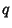elements. Then the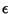-straight line complexity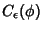of a function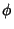is defined as the length of the shortest straight-line algorithm which computes a function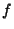such that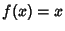is satisfied for at least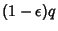elements of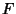. A functionis straight-line one way'' of range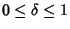ifsatisfies the properties:

1. There exists an infinite set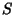of finite fields such thatis defined in every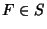andis One-to-One in every.

2. For everysuch that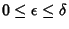,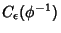tends to infinity as the cardinalityofapproaches infinity.

3. For everysuch that, the work function''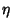satisfies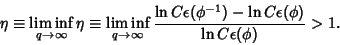It is not known if there is a one-way function with work factor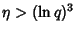.

References

Ziv, J. In Search of a One-Way Function'' §4.1 in Open Problems in Communication and Computation (Ed. T. M. Cover and B. Gopinath). New York: Springer-Verlag, pp. 104-105, 1987.